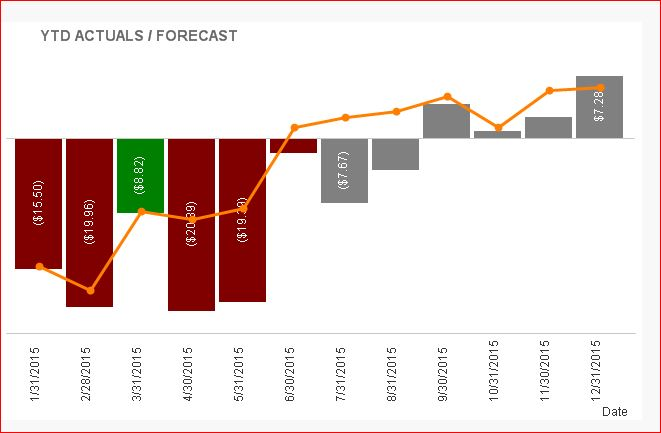# QlikView App Dev

Discussion Board for collaboration related to QlikView App Development.

Announcements
Join this live chat April 6, 10AM EST - QlikView to Qlik Sense REGISTER
cancel
Showing results for
Search instead for
Did you mean:Not applicable

## Conditional Format Date Range

I browsed the community and still am having trouble formatting values based on a given date range. For values that are less than the current month, I want to format them either red or green, while values greater than or equal to the current month colored grey. My current background color expression is as follows, any suggestions?

=
IF(
(
Sum({\$<[Sales Data.Metric]={'Direct Revenue'}, [FiscalMonth]={">=\$(=Max(FiscalMonth)<=\$(=Max(FiscalMonth)"}> [Sales Data.Actual])
-
Sum({\$<[Sales Data.Metric]={'Direct Revenue'}, [FiscalMonth]={">=\$(=Max(FiscalMonth)<=\$(=Max(FiscalMonth)"}>} [Sales Data.Plan])) < 0,
rgb(219,60,36),
IF(
(
Sum({\$<[Sales Data.Metric]={'Direct Revenue'}, [FiscalMonth]={">=\$(=Max(FiscalMonth)<=\$(=Max(FiscalMonth)"}> [Sales Data.Actual])
-
Sum({\$<[Sales Data.Metric]={'Direct Revenue'}, [FiscalMonth]={">=\$(=Max(FiscalMonth)<=\$(=Max(FiscalMonth)"}>} [Sales Data.Plan])) >= 0,
Green(),
rgb(128, 128, 128)))

21 RepliesMVP

Sorry forgot to do one thing:

=IF(
(
Sum({\$<[Sales Data.Metric]={'Direct Revenue'}, DN={">=\$(=Num(MonthStart(Date(Max(DN))))) <=\$(=Num(MonthEnd(Date(Max(DN)))))"}> [Sales Data.Actual])
-
Sum({\$<[Sales Data.Metric]={'Direct Revenue'}, DN={">=\$(=Num(MonthStart(Date(Max(DN))))) <=\$(=Num(MonthEnd(Date(Max(DN)))))"}>} [Sales Data.Plan])) < 0,
rgb(219,60,36),
IF(
(
Sum({\$<[Sales Data.Metric]={'Direct Revenue'}, DN={">=\$(=Num(MonthStart(Date(Max(DN))))) <=\$(=Num(MonthEnd(Date(Max(DN)))))"}>} [Sales Data.Actual])
-
Sum({\$<[Sales Data.Metric]={'Direct Revenue'}, DN={">=\$(=Num(MonthStart(Date(Max(DN))))) <=\$(=Num(MonthEnd(Date(Max(DN)))))"}>} [Sales Data.Plan])) >= 0,
Green(),
rgb(128, 128, 128)))Not applicable
Author

Still not working mateNot applicable
Author

BumpMVP

Hi Michael,

is it possible to post the sample qvw file? it would give the better understanding about your data.Not applicable
Author

Please see attachments, again, my end result is the following. I want to show YTD, Full Accumulation, with months < Today() formatted GREEN() if ACTUALS beat PLAN, is ACTUAL are under PLAN, RED(). IF Months are >= Today(), format them RGB(128, 128, 128).Example Chart: Conditional Coloring Based on Date RangeNot applicable
Author

Any luck?MVP

Hi,

if the YTD/ACTUALS is Full Accumulation, the value doesn't < YTD Plan value, right? (May be i understood wrongly)

See here..Just i tried to remove the Full Accumulation for YTD ACTUALS . It looks like thisif it is full accumulation, then YTD ACTUALS/FORECAST > YTD PLAN, right?

maybe somebody will help...Not applicable
Author

Yes, that bottom graph looks correct, however, the third bar (the one for 3/31/2015 should be GREEN(), with the remainder before 7/31/2015 colored RED()MVP

Hi Michael,

May be like this.I'm in the learning level. May be some expert will give the good idea.Master III

Hi Michhael,

I think you need an expression like the following. I am assuming that you have a fiscal date field in your data model..

You basically need to disregard all the other date releated selections like FiscalMonth,FiscalYear and just filter your data on your main date field(between Jan this year and year(Max(Your Date Field)) or Year(Today()).. Hope this helps

IF(

(Sum({\$<[Sales Data.Metric]={'Direct Revenue'},FiscalMonth =, FiscalYear =, FiscalDate ={">=\$(=YearStart(FiscalDate)<=\$(=Year(Max(FiscalDate))"}> [Sales Data.Actual])

-

Sum({\$<[Sales Data.Metric]={'Direct Revenue'}, FiscalMonth =, FiscalYear =, FiscalDate ={">=\$(=YearStart(FiscalDate)<=\$(=Year(Max(FiscalDate))">} [Sales Data.Plan])) < 0,

rgb(219,60,36),

IF(

(Sum({\$<[Sales Data.Metric]={'Direct Revenue'}, FiscalMonth =, FiscalYear =, FiscalDate ={"<=\$(=Max(FiscalDate)"}> [Sales Data.Actual])

-

Sum({\$<[Sales Data.Metric]={'Direct Revenue'}, FiscalMonth =, FiscalYear =, FiscalDate ={"<=\$(=Max(FiscalDate)"}>} [Sales Data.Plan])) >= 0,

Green(),

rgb(128, 128, 128)))